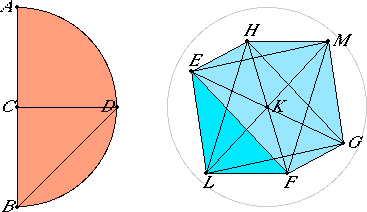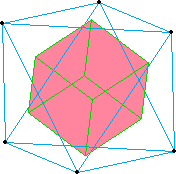# Proposition 14

To construct an octahedron and comprehend it in a sphere, as in the preceding case; and to prove that the square on the diameter of the sphere is double the square on the side of the octahedron.
I.11

Set out the diameter AB of the given sphere, bisect it at C, describe the semicircle ADB on AB, draw CD from C at right angles to AB, and join DB.

Set out the square EFGH, having each of its sides equal to DB, join HF and EG, set up the straight line KL from the point K at right angles to the plane of the square EFGH, and carry it through to the other side of the plane KM.I.3

Cut off KL and KM from the straight lines KL and KM respectively equal to one of the straight lines EK, FK, GK, or HK, and join LE, LF, LG, LH, ME, MF, MG, and MH.

I.47

Then, since KE equals KH, and the angle EKH is right, therefore the square on HE is double the square on EK. Again, since LK equals KE, and the angle LKE is right, therefore the square on EL is double the square on EK.

But the square on HE was also proved double the square on EK, therefore the square on LE equals the square on EH. Therefore LE equals EH. For the same reason LH also equals HE.

Therefore the triangle LEH is equilateral.

XI.Def.26

Similarly we can prove that each of the remaining triangles of which the sides of the square EFGH are the bases and the points L and M are the vertices, is equilateral, therefore an octahedron has been constructed which is contained by eight equilateral triangles.

It is next required to comprehend it in the given sphere, and to prove that the square on the diameter of the sphere is double the square on the side of the octahedron.

Since the three straight lines LK, KM, and KE equal one another, therefore the semicircle described on LM passes through E. And for the same reason, if, LM remaining fixed, the semicircle be carried round and restored to the same position from which it began to be moved, then it also passes through the points F, G, and H, and the octahedron will be comprehended in a sphere.

I say next that it is also comprehended in the given sphere.

I.4

For, since LK equals KM, while KE is common, and they contain right angles, therefore the base LE equals the base EM.

And, since the angle LEM is right, for it is in a semicircle, therefore the square on LM is double the square on LE.

Again, since AC equals CB, therefore AB is double BC. But AB is to BC as the square on AB is to the square on BD, therefore the square on AB is double the square on BD.

But the square on LM was also proved double the square on LE. And the square on DB equals the square on LE, for EH was made equal to DB. Therefore the square on AB equals the square on LM. Therefore AB equals LM.

And AB is the diameter of the given sphere, therefore LM equals the diameter of the given sphere.

Therefore the octahedron has been comprehended in the given sphere, and it has been demonstrated at the same time that the square on the diameter of the sphere is double the square on the side of the octahedron.

Q.E.F.

## Guide

Of the five regular polyhedra to be constructed in a sphere, the octahedron has the easiest construction. Relative to the center of the sphere K, the lines to the six verices KE, KF, KG, KH, KL, and KM form three mutually perpendicular diameters. Also, the 12 sides group into three groups of four lines, each group forming the vertices of a square—EFGH, EMGL, and FMHL.

Since the center of each square is the center of the sphere, therefore two sides, EF and FG, along with the one diameter EG of the octahedron form a 45°-45°-90° triangle. Thus, the square on the diameter of the sphere is twice the square on the side of the octahedron.

#### Coordinates for the vertices of the octahedron

If the sphere circumscribing the octahedron is the unit sphere, then a natural coordinate system to impose would have the three coordinate axes be the three perpendicular diameters. Then the points a unit distance from the origin are the six vertices of the octahedron, namely,

(1,0,0)   (–1,0,0)   (0,1,0)   (0,–1,0)   (0,0,1)   (0,0,–1)

#### Duals of the regular polyhedra

As will be shown in proposition XIII.18, there are exactly five regular polyhedra. The accompanying table lists these five polyhedra along with the numbers of the their faces, edges, and vertices. Their names are taken from the number of their faces, except, of course, the cube, which otherwise would be called a hexahedron.

PolyhedronFacesEdgesVertices
tetrahedron464
octahedron8126
cube6128
icosahedron203012
dodecahedron123020

Note that there are two pairs of polyhedra in this table where the numbers are related. One pair is the octahedron and cube, the other is the icosahedron and dodecahedron. For these pairs the number of faces of one of the pair equals the number of vertices of the other, and both of the pair have the same number of edges. These are the pairs of “duals.” The numbers for the tetrahedron indicate that it dual to itself.

 We can see the correspondence between the parts of one of these polyhedra and the parts of its dual. Consider the octahedron. Place a point in the circumcenter of each of the eight faces. Connect two of these points if the faces that contain them share an edge. For each of the six vertices of the octahedron, connect the four circumcenters of the adjacent faces to make a square. What results is a cube with six vertices, 12 edges, and eight faces. An analogous construction for the cube yields an octahedron. Likewise the constructions for the icosahedron and dodecahedron yield each other, and the construction for a tetrahedron yields another tetrahedron. (Spin the figure by dragging around the orange vertices.)#### Use of this construction

Constructing this octahedron is an end in itself. The construction is also used in proposition XIII.18 where the five regular polyhedra are compared.